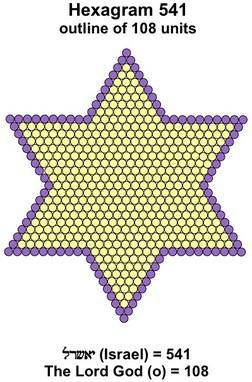Thread: 37 View Single Post22-10-2016, 08:30 AM #8 markgobell Senior Member   Join Date: Dec 2011 Posts: 2,987 Likes: 1,111 (659 Posts)The numbers 37, 73 and 666 & the Star of David or Seal of Solomon There's quite a bit on t'interwebs about the significance of 37 ( P12 ) and 73 ( P21 ) vis the Star of David or Seal of Solomon. My working hypothesis is that the current Star of David = 37 dots = the minimum dots required to build the first proper Star of David represents the current state of Israel.The next largest Star of David requires 73 dots which may well represent the goal of an expanded Greater Israel or maybe that expansion will be incremental up to the Star of David with 541 ( P100 ) dots, which happens to be the gematria value of Israel.37 and 73 can also be derived from the second proper 73 dot Star of David thus : http://www.biblegematria.com/pearls-of-wisdom.html37 can also be derived from any size Star of David using another method : 1. Count the number of line intersections = the points where the lines either meet or cross each other = 12 2. Count the number of external lines = the two sides of each of the six triangles = 12 3. Count the number of internal lines = the faces of the internal hexagon = 6 4. Count the number of spaces = 6 triangles plus 1 hexagon = 7 12 + 12 + 6 + 7 = 37 So, regardless of the actual size of each Star of David, using this method will always produce the number 37 ( P12 = P( 3x2x2 ) = P( 4+4+4 ) ) Some resources also explain the geometric derivation of the 666 triplet from each triangle ( as above and so below ? ) within the Star of David, thus yiedling 666 x6 in the hexagram. From my preferred link posted above : http://members.home.nl/frankcolijn/f...r_of_David.htmFrom biblegematria.com Here, the 541 ( P100 ) hexagram ( Israel ) is surrounded by 6 convex hexagons, each with 666 dots ( 6x 666 = 3996 = 444 x3 x3 )Perimeter of each segment of the wisdom star is 108 - the Israel star has a perimeter of 108, as does each 666 convex hexagon. Here the same hexagram is divided differently, yielding 3 Alpha Omega segments, each with a perimiter of 216 dots, which yields another 666 motif thus : 216 = 6x6x6 >666Perimeter of each Alpha Omega segment is 216, the same as the perimeter of the Genesis 1:1 triangle. Each segment consists of 1332 dots = 2x 666 and 3x 444 666 Hidden in Gen 1:1, Genetic Code=Star of David (37) Gen 1:1=Star of David=37=12 Tribes of Israel-Gematria etc. It is no surprise to me for reasons explained above, that the number 37 is frequently used to form the root of many of the date relationships between world events. Whether that 37 is implicit but hidden as in the use of the 3 digit rep-digits ( 111 to 999 ), or when 37 and it's multiples are used as natural numbers. The hidden or occult value of the Prime Number 37 lies in the use of it's Prime Ordinal or Prime Sequence Number P12 also explained above. This occult 12 may well represent the 12 Biblical tribes. Note also that 12 is the hidden or occult basis for the Prime Number 911 ( P156 ) as explained here. The Number: 911 I am not sure if I am correct in further defining these 12's by deriving the numerological / concatenated numbers 322 and 444 by breaking down 12 into it's factors ( 3x2x2 > 322 > Skull & Bones > 33° World Masonry ) and or the triplet derived from it's idential sum components ( 4+4+4 > The Perfect Masonic Number ). Note also that there is speculation about whether the two blue lines on the flag of Israel which delineate it's Star of David, represent the two rivers, the Nile and the Euphrates which are said to have delineated the Biblical borders of Israel and therefore the goal of an expanded Greater Israel. This notion has been dismissed as the "Nile to Euphrates" conspiracy theory" which imo, probably means that it may well be true.. Last edited by markgobell; 22-10-2016 at 10:17 AM.